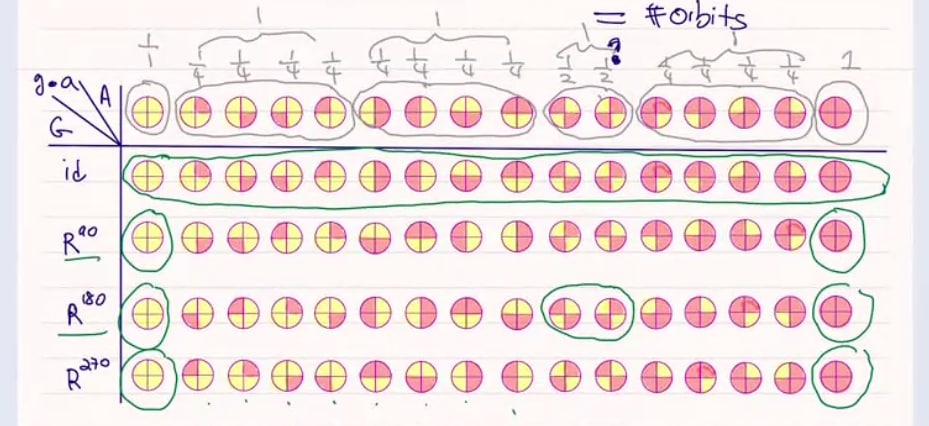Burnside’s Lemma
Statement
Burnside’s lemma, also called the lemma that is not Burnside’s, gives an expression for the number of orbits formed by a group action. Take a finite group $G$ acting on a finite set $A$. The number of orbits in this group action is given by $\frac 1 { \lvert G \rvert } \sum_{g \in G}{\lvert A^g \rvert}$ where $A^g$ denotes all those elements $a \in A$ fixed by $g$.
Proof
Take finite $G$ acting on finite $A$. Let $F$ denote the set of pairs consisting of a $g \in G$ and an $a \in A$ fixed by $g$; that is, $F = \{ (g, a) \in G \times A \mid g \bull a = a \}$ The proof essentially proceeds by giving three different ways to count this set $F$. Way 1. We can decompose $F$ along elements $g \in G$ as $F = \bigcup_{g \in G} \{ a \in A \mid g \bull a = a \} = \bigcup_{g \in G} A^g$ giving rise to the identity $\lvert F \rvert = \sum_{g \in G}{\lvert A^g \rvert}$ Way 2. We can decompose $F$ along elements $a \in A$ as $F = \bigcup_{a \in A} \{ g \in G \mid g \bull a = a \} = \bigcup_{a \in A} \text{stab}(a)$ where $\text{stab}(a)$ denotes the stabilizer of $a$. This gives rise to the identity $\lvert F \rvert = \sum_{a \in A}{\lvert \text{stab}(a) \rvert}$ Way 3. Similar to way 2, we can decompose $F$ along elements $a \in A$; however, we will additionally group these elements by orbit: $F = \bigcup_{\text{orbit } O} \bigcup_{a \in O} {\text{stab}(a)}$ This is possible because the orbits form a partition of $A$. This gives rise to the identity $\lvert F \rvert = \sum_{\text{orbit } O} \sum_{a \in O} {\lvert \text{stab}(a) \rvert}$ Now note that for any given orbit $O$ we have *
Adding a reference here to the orbit-stabilizer theorem, since references within math typesetting are not recognized =(
\begin{align*} & \sum_{a \in O} {\lvert \text{stab}(a) \rvert} \\ &= \sum_{a \in O} {\lvert G \rvert \cdot \lvert \text{orbit(a)} \rvert^{-1}} &&\text{orbit-stabilizer theorem} \\ &= \sum_{a \in O} {\lvert G \rvert \cdot \lvert O \rvert^{-1}} && a \in O \implies \text{orbit}(a) = O \\ &= \lvert O \rvert \cdot \left( {\lvert G \rvert \cdot \lvert O \rvert^{-1}} \right) && \text{sum body invariant wrt sum variable} \\ &= \lvert G \rvert \end{align*} Plugging this back into the identity, we get $\lvert F \rvert = \sum_{\text{orbit }O} \lvert G \rvert$ In other words, when decomposing $F$ along orbits, each orbit $O$ contributes exactly $\lvert G \rvert$ to the final sum. Now we will rewrite this one final time as $\lvert F \rvert = \lvert G \rvert \cdot \#\text{ of orbits}$ Combining the three established identities, we get $\lvert F \rvert = \sum_{g \in G} {\lvert A^g \rvert} = \sum_{a \in A} {\lvert \text{stab}(a) \rvert} = {\lvert G \rvert} \cdot \#\text{ of orbits}$ Picking out the second and fourth expressions, and dividing both by $\lvert G \rvert$, we get $\frac 1 {\lvert G \rvert} \sum_{g \in G} {\lvert A^g \rvert} = \#\text{ of orbits}$ which is exactly the statement of Burnsides’s lemma; done! rk
Note that we did not actually end up using counting method #2; it’s a stepping stone to method #3 as well as a footnote of yet another way to compute $\lvert F \rvert$.
As a final remark, I include the following possibly-helpful diagram which my professor made. In this diagram, we have $G = \mathbb Z / 4$ acting by rotation on $A = \mathbf 2^4$ (length-4 bitstrings). Along the y-axis we have elements $g \in \mathbb Z / 4$, along the x-axis we have elements $a \in \mathbf 2^4$, and in cells we have $g \bull a$.Elements of $F$ are circled in green, and orbits are circled in grey. Our first way of counting $F$, which is along elements $g \in G$, is visualized here by summing green-circled elements along rows. Likewise, our second way of counting $F$, along elements $a \in A$, is a summation along columns. The third way, along orbits, is visualized by summing along columns, but grouping the columns into orbits. Note that each orbit contributes exactly $4 = \lvert G \rvert$ elements!
Example application
We can use Burnside’s Lemma in order to aid counting the number of cyclic bitstrings of a given size. For illustrative examples, let’s first consider the specific case of cyclic bitstrings of length 3. Let $\mathbf 2$ denote the set $\{0,1\}$; then we can write the set of (non-cyclic) bitstrings as $\mathbf 2^3$. Organized by length, $\mathbf 2^3$ looks like this:
Define an equivalence relation $\sim$ by identifying bitstrings which are rotations of each other. Then the set of cyclic bitstrings of length 3 is given by $\mathbf 2^3 / \sim$, which we can visualize as so:
Separately, consider the group $\mathbb Z / 3$ acting on $\mathbf 2^3$ by rotation; that is, where $\bar n \bull b$ is $b$ rotated $n$ times. Such a group action exhibits the following orbits:
Note that the orbits of this group action correspond exactly to the equivalence classes of $\mathbf 2^3 / \sim$. As such, the number of equivalence classes (and thus the number of cyclic bitstrings) is given by Burnside’s Lemma as \begin{align*} & \frac 1 { \lvert \mathbb Z/3 \rvert } \sum_{r =\bar 0, \bar 1, \bar 2} \left\lvert \left( \mathbf 2^3 \right)^r \right\rvert \\ &= \frac13\left( 8 + 2 + 2 \right) \\ &= 4 \end{align*} Further, this approach generalizes nicely rk! In general, the number of cyclic bitstrings of length $\ell$ is given by
Generalizing to longer bitstrings is really the utility of using Burnside’s lemma here; in the specific case of length-3 bitstrings we could have just stopped after constructing the diagram for $\mathbf 2^3/\sim$ by noting that our answer is $\lvert \mathbf 2^3/\sim \rvert = 4$.
$\frac 1 {\lvert \mathbb Z/\ell \rvert} \sum_{r=\bar 0, \dots, \overline {\ell - 1}} \left\lvert \left( \mathbf 2^\ell \right)^r \right\rvert$

Referenced by: